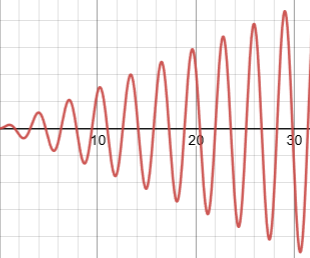## Sunday, October 17, 2021

### Musical Strings 2: UNIZOR.COM - Physics4Teens - Waves - Transversal Waves

Notes to a video lecture on http://www.unizor.com

Musical Strings 2

In the previous lecture we considered the model of a musical string as a point-mass m between two identical springs of the length (L+l)/2 each (where L is a neutral length of the string we are modeling and l is a stretch to create initial tension) with a coefficient of elasticity k.Plucking a string is, therefore, modeled as an initial displacement of a point-mass vertically by y(0)=a.

The result of the analysis of forces acting on a point-mass between these springs, when it performs transverse oscillations, was a differential equation
y"(t) + 2·(κ/m)·y(t)·
·
[1−L/(L+l)²+4y²(t)] = 0

Let's examine this model from the energy standpoint.
By lifting a point-mass initially by distance y(0)=a, we perform some work against elastic forces of two springs. The forces are variable and depend on how much we stretch the springs. Let's take coordinate y as our argument. Then, as explained in the previous lecture, the forces of two springs acting along the Y-axis against our motion total to
F↓↓(y) = −2·κ·y·
·
[1 − (L/2) /((L+l)/2)²+y²] =
= −2·κ·y·
[1 − L /(L+l)²+4y²]
Incidentally, it's easy to see that an expression in [...] is always positive, therefore the sign of the force is always opposite to the sign of a displacement y.

On an interval from y to y+dy of the infinitesimal length dy we can assume the constant force, as described above.
Therefore, the work we perform on that infinitesimal interval by going against the force F↓↓(y) is
dW = −F↓↓(y)·dy

We can now calculate the total work performed by displacing our point-mass by distance y(0)=a by integrating the above expression from y=0 to y=a.
W[0,a] = −[0,a]F↓↓(y)·dy =
=
[0,a]2·κ·y·
·
[1−(L/2) /((L+l)/2)²+y² ]·dy =
=
[0,a]2·κ·y·
·
[1−L/(L+l)²+4y² ]·dy

This integral can be calculated.
W[0,a] = 2·κ·[y²/2 −
− (L/4)·√(L+l)²+4y²
] |[0,a] =
= 2·κ·
[a²/2 −
− (L/4)·√(L+l)²+4a² +
+ L·(L+l)/4
] =
= κ·
[a² − (L/2)·√(L+l)²+4a² +
+ L·(L+l)/2
]

Just to check, if a=0, the work performed is
W[0,0] =
= κ·
[0² − (L/2)·√(L+l)²+0² +
+ L·(L+l)/2
] = 0
as it is supposed to be.

Analysis of the potential energy W(a) as a function of the initial displacement a shows that it resembles the parabola with a graph like this: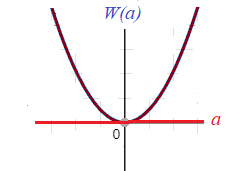So, potential energy is always positive, as it should be, and grows with initial displacement a growing to positive or negative direction (up or down on a picture of a point-mass between two springs above).

At any displacement point y from the neutral position a point-mass m has certain potential and kinetic energy.
Its potential energy W(y), as a function of displacement y, looks exactly the same as the one we calculated at the initial position, when y=a, which yields
W(y) =
= κ·
[y² − (L/2)·√(L+l)²+4y² +
+ L·(L+l)/2
]

Obviously, this formula is valid only for −a ≤ y ≤ a.
The difference between W[0,a] and W(y) is the kinetic energy our point-mass possesses because it moves towards the neutral position, that is
K(y)=m·(y')²/2.

Therefore, we have an equation, which is a differential equation for a displacement y(t) as a function of time t (in many cases we will drop (t) for brevity).
W(y) + K(y) = W[0,a]

The differential equation for displacement y(t) is
κ·[y² − (L/2)·√L+l)²+4y² +
+ L·(L+l)/2
] + m·(y')²/2 =
= κ·
[a² − (L/2)·√(L+l)²+4a² +
+ L·(L+l)/2
]

Simplifying...
y² − (L/2)·√(L+l)²+4y² +
+ (m/κ)·(y')²/2 =
= a² − (L/2)·√(L+l)²+4a²

or
(m/2κ)·(y')² + y² −
− (L/2)·√(L+l)² + 4y² =
= a² − (L/2)·√(L+l)²+4a²

This is a different differential equation that describes the transverse oscillations of a point-mass between two springs than that described in the previous lecture.
It contains only the first derivative of a displacement y(t).

Interestingly, though not surprisingly, this equation is not really different.
If two functions are equal, their derivatives are also equal. Take a derivative from both sides of an equation above:
(m/2κ)·2y'·y" + 2y·y' −
− (L/2)·8y·y' /2√(L+l)² + 4y² =
= 0

or
(m/2κ)·y'·y" + y·y' −
− L·y·y' /(L+l)² + 4y² =
= 0

Now we can cancel y'(t) getting
(m/2κ)·y" + y −
− L·y /(L+l)² + 4y² = 0

or
(m/2κ)·y" + y·
·
[1 − L /(L+l)² + 4y²] = 0
or
y" + (2κ/m)·y·
·
[1 − L /(L+l)² + 4y²] = 0
which is exactly the same as the equation derived in the previous lecture.

## Friday, October 8, 2021

### Musical Strings 1: UNIZOR.COM - Physics4Teens - Waves - Transversal Waves

Notes to a video lecture on http://www.unizor.com

Musical Strings 1

Our first example of transverse oscillations was the one with a rope, one end of which we shake up and down, with another end free.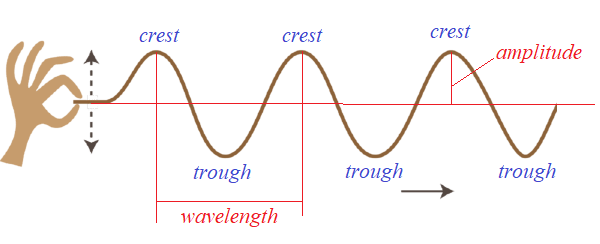(open this picture in a new tab of your browser by clicking the right button of a mouse to better see details)

Now we will consider another type of transverse oscillations that is used in string musical instruments, like a guitar or violin.

The real oscillations of a violin string are quite complex, so we will have to rely on some simplified model that allows analytical approach.
So, here is the first really simple model of an oscillating string.

String of a violin or a guitar has two ends fixed and it oscillates when it's plucked.
The mechanism of plucking involves stretching a string at some middle point perpendicularly to a string direction and letting it go.

It is important to notice that real guitar or violin strings are initially stretched to create some tension, without which there will be no pleasant sound.
Also important is that real strings have a high coefficient of elasticity, so even a small stretch creates significant tension force.

In our simplified model we replace a string with two identical weightless springs of combined length L in a neutral (not stretched, nor squeezed) state, but stretched by a combined increment l to create initial tension, and a point-mass m fixed in the middle between the springs.
So, the neutral length of each spring is L/2 and the length increment of each spring, as it is initially stretched, is l/2.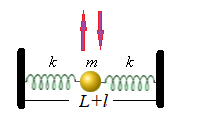According to the Hooke's Law, the tension caused by initial stretch of each spring is
T = k·l/2
The initial tension forces of both springs act on a point-mass in the middle with equal magnitude and opposite directions and neutralize each other.

Plucking the point-mass in the upward direction, thus further stretching both springs it's attached to, and letting it go resembles the plucking of a string on a musical instrument, like a guitar or a violin.
The point-mass will start oscillating in the vertical direction, performing transversal oscillations.

This simplified model allows for analytical approach to find the differential equation of this type of oscillation.
Let's state in advance that the differential equation obtained will not yield to an easy solution, so we will just explain how to get to it, but then switch to another way to approach this problem.

Let's examine the forces acting on our point-mass after we lift it by the initial distance a and let it go, so it's performing vertical oscillations with y(t) being a deviation from the initial position at time t.

The initial condition of this motion is, therefore,
y(0) = a
y'(0) = 0

Assume that at time t the vertical deviation of a point-mass from its initial position is y(t). Then the two forces from two stretched springs acting on this point-mass are in red on a picture below.The forces can be evaluated using the Hooke's Law.
The neutral (unstretched) length of each spring is L/2, but it's initially stretched by l/2. The new stretched length is ((L+l)/2)²+y²(t). The force is proportional to elongation of a spring with a coefficient κ - spring's elasticity.
Therefore, each force by absolute value equals to
|F(t)| =
= κ·
[((L+l)/2)²+y²(t) − L/2]

We need to account only for vertical components of these two forces since horizontal components will act against each other and neutralize each other.
If each spring makes an angle θ with horizontal line, the absolute value of the vertical component of each force is
|F(t)| = |F(t)|·sin(θ)
In terms of y(t) the vertical component of both forces is
F(t) =
= −|F(t)|·y(t) /((L+l)/2)²+y²(t)

Since vertical components from both springs are added together, the total force F↓↓(t) acting on a point-mass is double the above expression, which should be simplified as follows.
F↓↓(t) = m·y"(t) =
= −2·|F(t)|·
·y(t) /(L+l/2)²+y²(t) =
= −2·κ·y(t)·
·
[1 − (L/2) /(L+l/2)²+y²(t)]

This gives us a differential equation of a transverse movement of a point-mass between two springs
m·y"(t) = −2·κ·y(t)·
·
[1 − (L/2) /((L+l)/2)²+y²(t)]

y"(t) + 2·(κ/m)·y(t)·
·
[1−(L/2)/((L+l)/2)²+y²(t)] = 0

If we consider the above equation as it is, it's too complex to get a nice solution. In cases like this physicists usually resort to reasonable approximations.

In this case we can safely assume that vertical deviation y(t) is small relatively to a the length L and, therefore, adds an insignificant amount to a radical in the denominator. So, let's just drop it from the equation, getting after a series of trivial steps a simpler differential equation
(a) y"(t) + 2·(κ/m)·y(t)·
·
[1−(L/2)/((L+l)/2)²] = 0
(b) y"(t) + 2·(κ/m)·y(t)·
·
[1−(L/2)/((L+l)/2)] = 0
(c) y"(t) + 2·(κ/m)·y(t)·
·
[1−L/(L+l)] = 0
(d) y"(t) + 2·(κ/m)·y(t)·
·l /(L+l) = 0

(e) y"(t) + ω²·y(t) = 0
where
ω² = 2·κ/[m·(1+L/l)]

The final equation is a familiar differential equation that describes harmonic oscillations with angular frequency ω.

From the expression
ω² = 2·κ/[m·(1+L/l)]
we see that greater initial stretch of our springs l contributes to higher angular frequency of oscillations, which corresponds to our experience with real strings - more initial tension applied to a string results in a higher tone of vibrations.

Considering initial conditions
y(0) = a and y'(0) = 0,
the vertical displacement of our point-mass between two springs will be
y(t) = a·cos(ωt)
where ω is evaluated above.

## Sunday, September 12, 2021

### Transverse Waves: UNIZOR.COM - Physics4Teens - Waves

Notes to a video lecture on http://www.unizor.com

Transverse Wave

We are familiar with longitudinal waves, like sound waves in the air.
Their defining characteristic is that molecules of air (the medium) are oscillating along the direction of the wave propagation, which, in turn, causing oscillation of pressure at any point along the direction of wave propagation.

Consider a different type of waves.
Take a long rope by one end, stretching its length on the floor. Make a quick up and down movement of the rope's end that you hold.
The result will be a wave propagating along the rope, but the elements of rope will move up and down, perpendicularly to the direction of waves propagation.(open this picture in a new tab of your browser by clicking the right button of a mouse to better see details)

These waves, when the elements of medium (a rope in our example) are moving perpendicularly to a direction of waves propagation, are called transverse.

Such elementary characteristics of transverse waves as crest, trough, wavelength and amplitude are clearly defined on the picture above.

Some other examples of transverse waves are strings of violin or any other string musical instrument.

Interesting waves are those on the surface of water. They seem to be transverse, but, actually, the movement of water molecules is more complex and constitutes an elliptical kind of motion in two directions - up and down perpendicularly to waves propagation and back and forth along this direction.

Our first problem in analyzing transverse waves is to come up with a model that resembles the real thing (like waves on a rope), but yielding to some analytical approach.

Let's model a rope as a set of very small elements that have certain mass and connected by very short weightless links - sort of a long necklace of beads.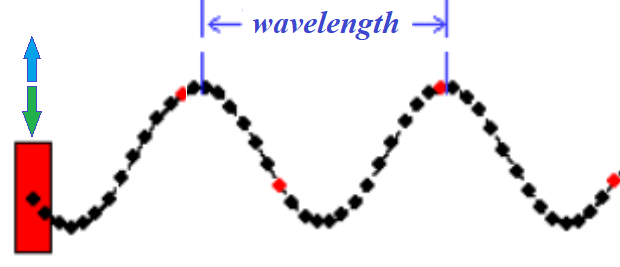Every bead on this necklace is a point-object of mass m, every link between beads is a solid weightless rod of length r.
Both mass of an individual bead m and length of each link r are, presumably, very small. In theory, it would be appropriate to assume them to be infinitesimally small.

Even this model is too complex to analyze. Let's start with a simpler case of only two beads linked by a solid weightless rod.
Our purpose is not to present a complete analytical picture of waves, using this model, but to demonstrate that waves exist and that they propagate.

Consider the following details of this model.
Two identical point-objects α and β of mass m each on the coordinate plane with no friction are connected with a solid weightless rod of length r.

Let's assume that α, initially, is at coordinates (0,−A), where A is some positive number and β is at coordinates (a, −A).
So, both are at level y=−A, separated by a horizontal rod of length r from x=0 for α to x=a for β.

We will analyze what happens if we move the point-object α up and down along the vertical Y-axis (perpendicular to X-axis), according to some periodic oscillations, like
yα(t) = −A·cos(ω·t)
where
A is an amplitude of oscillations,
ω is angular frequency,
t is time.
Incidentally, for an angular frequency ω the period of oscillation is T=2π/ω.
So, the oscillations of point α can be described as
yα(t) = −A·cos(2π·t / T)

Point α in this model moves along the Y-axis between y=−A and y=A.
It's speed is
y'α(t) = A·ω·sin(ω·t) =
= A·(2π/T)·sin(2π·t/T)

The period of oscillations is, as we noted above
T = 2π/ω

During the first quarter of a period α moves up, increasing its speed from v=0 at y=−A to v=A·ω at the point y=0, then during the next quarter of a period it continues going up, but its speed will decrease from v=A·ω to v=0 at the top most point y=A.
During the third quarter of a period α moves down from y=A, increasing absolute value of its speed in the negative direction of the Y-axis from v=0 to the same v=A·ω at y=0, then during the fourth quarter of a period it continues going down, but the absolute value of its speed will decrease from v=A·ω to v=0 at the bottom y=−A.

Positions of objects α and β during the first quarter of a period of motion of α at three consecutive moments in time are presented below.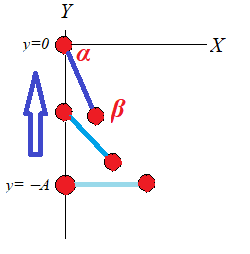As the leading object α starts moving up along Y-axis, the led by it object β follows it, as seen on a picture above.

Let's analyze the forces acting on each object in this model.
Object β is moved by two forces: tension from the solid rod Tβ(t), directed along the rod towards variable position of α, and constant weight Pβ.
Object α experiences the tension force Tα(t), which is exact opposite to Tβ(t), the constant weight Pα and pulling force Fα(t) that moves an entire system up and down.

There is a very important detail that can be inferred from analyzing these forces.
The tension force Tα(t), acting on object α, has vertical and horizontal components from which follows that pulling force Fα(t) cannot be strictly vertical to move object α along the Y-axis, it must have a horizontal component to neutralize the horizontal component of Tα(t).

This can be achieved by having some railing along the Y-axis that prevents α to deviate from the vertical path. The reaction of railing will always neutralize the horizontal component of the Tα(t). Without this railing the pulling force Fα(t) must have a horizontal component to keep α on the vertical path along the Y-axis.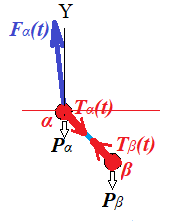If α and β are the first and the second beads on a necklace, we can arrange the railing for α. But, if we continue our model and analyze the movement of the third bead γ attached to β, there can be no railing and the horizontal component of the tension force Tβ(t) will exist and will get involved on some small scale.

All-in-all, transverse motion is not just movement of components up and down perpendicularly to the wave propagation, it is also a longitudinal motion of these components, though not very significant in comparison with transverse motion and often ignored in textbooks.

The really obvious reason for transverse motion to involve a minor horizontal movement in addition to a major vertical one is that you cannot lift up a part of a horizontally stretched rope without a little horizontal shift of its parts as well, because a straight line is always shorter than a curve.

Let's now follow the motion of β as α periodically moves up and down, starting at point (0,−A), according to a formula
yα(t) = −A·cos(ω·t)
with its X-coordinate always being equal to zero.

Since position of α is specified as a function of time and the length of a rod connecting α and β is fixed and equal to r, position of β can be expressed in term of a single variable - the angle φ(t) from a vector parallel to a rod directed from α to β and the positive direction of the Y-axis.
At initial position, when the rod is horizontal, φ(0)=π/2.

Then coordinates of β are:
xβ(t) = r·sin(φ(t))
yβ(t) = yα(t) + r·cos(φ(t))

During the first quarter of a period α moves up from y=−A to y=0, gradually increasing its speed and pulling β by the rod upwards.

While β follows α up, it also moves closer to the Y-axis. The reason for this is that the only forces acting on β are the tension force along the rod and its weight.
Weight is vertical force, while tension acts along the rod and it has vertical (up) and horizontal (left) components. Assuming vertical pull is sufficient to overcome the weight, β will be pulled up and to the left, closer to the Y-axis.

If this process of constant acceleration of α continued indefinitely, β would be pulled up and asymptotically close to the Y-axis. Eventually, it will just follow α along almost the same vertical trajectory upward.
The angle φ(t) in this process would gradually approach π (180°).

With a periodic movement of α up and down the Y-axis, the trajectory of β is much more complex.
Let's analyze the second quarter of the period of α's oscillations, when it moves from y=0 level, crossing the X-axis, to y=A.

After α crosses the X-axis it starts to slow down, decelerate, while still moving up the Y-axis.
As α decelerates in its vertical motion up, the composition of forces changes.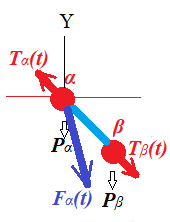Now β will continue following α upwards, but, instead of being pulled by the rod, it will push the rod since α slows down.

That will result in the force Tα(t), with which a rod pushes α, to be directed upwards and a little to the left, as presented on the above picture.
Also Tβ(t), the reaction of the rod onto β, will act opposite to a tension during the first quarter of a period. Now it has a vertical component down to decelerate β's upward motion and a horizontal component to the right.

The latter will cause β to start moving away from the Y-axis, while still going up for some time, slowing this upward movement because of opposite force of reaction of the rod.

When α reaches level y=A at the end of the second quarter of its period, it momentarily stops.
The behavior of β at that time depends on many factors - its mass m, amplitude A and angular frequency ω of α's oscillations, the length of the rod r.
Fast moving leading object α will usually result in a longer trajectory for β, while slow α will cause β to stop sooner.

During the third quarter of its period α starts moving downward, which will cause β to follow it, but not immediately because of inertia. Depending on factors described above, β might go up even higher than α. In any case, the delay in β's reaching its maximum after α has completed the second quarter of a period will exist, that would cause β to reach its maximum later than α.

The delay between reaching its maximum height by β is the source of a visual effect that call waves. Every subsequent bead on a necklace will reach its top height a little later than the previous.

Assuming that α accelerates faster than g on its way down during the third quarter of a period, α will pull β down and closer to the Y-axis. Situation will be similar to the first quarter of a period, when α accelerates up.
Then during the fourth quarter α will slow down to full stop, while inertia and weight move β faster down, so it will have to make a small circle around the last level of α before a new period starts, and α pulls β again up.

The above relatively complicated description of the trajectory of β is supposed to serve as an explanation of the fact that this trajectory represents not simply an up and down motion of beads of a necklace in our model of the transverse oscillations, but is much more complex motion that involves both transverse and longitudinal oscillations with former playing a major role, while latter, however small, is still an important part of a motion.

## Saturday, August 7, 2021

### Reflection of Waves: UNIZOR.COM - Physics4Teens - Waves - Waves in Medium

Notes to a video lecture on http://www.unizor.com

Reflection Waves

Let's consider the same simple device as in the previous lecture - a cylinder of relatively small diameter with air and a piston in it, except this time we will examine what happens at the opposite to a piston end of a cylinder.Let's give a single short and quick push to a piston down. As a result, a single parallel to a piston wave of a higher pressure air will start its way down a cylinder with certain speed v that depends on properties of air, its temperature, initial pressure etc.

When this wave reaches the bottom of a cylinder, a thin layer of higher pressure air will develop there. Since the bottom of a cylinder prevents a wave to go farther, the higher pressure air will release its pressure back to a layer above it. This constitutes a reflection of the wave.

After such a reflection the wave will go to the opposite direction (upward on the picture) until it reaches the top, where piston is positioned. At this moment, since piston is not moving, it will play the same role as a bottom of a cylinder before, it will reflect the wave.

Ideally, this reciprocal movement of a wave can continue, it will be reflected from both ends of a cylinder indefinitely.
Practically, the energy of the initial push of a piston will gradually dissipate into more intense movement of the air molecules, which means that the temperature of the air will increase slightly.

Consider now a harmonic oscillation of a piston. It produces pressure waves in the air propagated downward. But now we know that they reflect from the bottom of a cylinder and propagate upwards.
This results in a very complex superposition of two sets of waves, one going downwards and another upwards.
There is, generally speaking, a shift in phase between these two sets of waves that depends on the speed of wave propagation and the length of a cylinder.

The resulting picture of air pressure will look quite chaotic and far from harmonic oscillations of a piston. Yet, it's just a simple superposition of waves moving in two opposite directions.

Imagine a complexity of a sound distribution in an opera house with air pressure waves, originated by a person singing on a stage, reflected from all hard surfaces and mixing in time and space with other waves. Unless special design measures are taken, the quality of a sound will be very low.

Even in such a simple case as recording these lectures we had to use special directional microphone that suppresses sounds from all directions except the main one directed onto a primary source of sound. In addition, to weaken the reflection, we open closets on the opposite wall to weaken the strength of reflected sound waves.

Let's analyze the reflection of a simple flat wave from a surface that is not perpendicular to the wave front, keeping in mind the Law of Reflection, that is, the angle of incidence equals to the angle of reflection.
Assume that our flat wave consists of only two molecules of air a and b that hit a surface AB at 45° angle.
A thin line between these molecules represents a wave's front, which is perpendicular to their trajectories.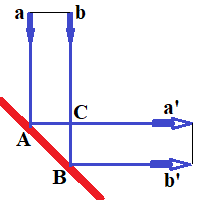From a picture above and some elementary geometry it's obvious that reflected wave will be exactly as original, except its direction will be perpendicular to the original's one.
Indeed, a and b move on parallel trajectories with the same speed, covering the same distance in equal times. So, molecule a will come to point C at the same time as molecule b reaches point B. After that a and b continue their parallel movement and the new wave's front is still perpendicular to molecules' trajectories.

Even if the angle of a reflecting surface is not 45° to a direction of the original wave, the reflected wave will still be a flat wave with only direction changed.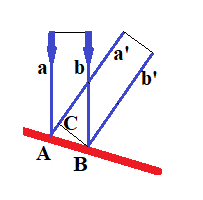It's easy to prove that molecule a will reach point C at the same time as molecule b comes to point B. After that both molecules continue their straight movement with a wave front perpendicular to their parallel trajectories.

We should not think about waves and their reflections as a flow of the same molecules toward a reflective surface, reflecting from it and continuing the movement. Different crests of waves have different molecules. But the movement of one molecule is transferred to its neighbor in the direction of a wave propagation as if they are connected with tiny springs.

Another good comparison of this process is the balls on a billiard table. When a ball hits another ball straight on, it stops and the other ball continues the movement in the same direction. If the hit is not exactly straight on, the impulse of a hitting ball will be distributed among two balls, but their sum will be equal to the original impulse.
So, the energy and impulse are transferred in some direction without carriers of this energy and impulse actually moving all the way to the end of a road, but rather by transferring this energy and impulse to other carriers, like in the relay race.

Let's return back to a case when a flat solid surface is perpendicular to a direction of propagation of the flat waves, like a bottom of a cylinder described above.
As mentioned above, reflected from this obstacle waves will be reflected in the opposite direction, thus interfering with original waves.
Both oscillations, downwards and upwards, have exactly the same period T and wavelength λ. Their speeds v are equal by absolute value, but oppositely directed.

Depending on difference in phase, superposition of these two waves can produce different interesting effects.

An oscillation of air in a cylinder, produced by an oscillation of a piston, is just a periodic higher and lower concentration of molecules of air (that is, higher or lower pressure) with the waves of high concentration moving from a piston down a cylinder with some speed.

Add to this picture reflected waves formed at the opposite end of a cylinder - waves of higher and lower concentration of molecules of air (that is, higher or lower pressure) moving up the cylinder.

Both processes mix together in a superposition. When a wave crest from a piston reaches some layer of air, it pushes this layer down.
When the opposite reflected wave crest reaches the same layer, it pushes this layer up.
With proper difference in phase, that depends on the length of cylinder relatively to a wavelength λ, there might be a situation when the same layer of air in a cylinder is simultaneously pushed down by a wave crest from a piston and up by a reflected wave crest. As a result, this layer will be squeezed from both ends, the pressure in this layer will become higher.

For similar reasons the layer on a distance λ/2 (half a wavelength) from the one that is squeezed from both sides will be thinner, and the pressure inside this layer will be lower.

In T/2 (half a period) time the area that used to be with higher concentration of molecules will become thinner, as its molecules will move in opposite directions, thus making areas on a distance of half a wavelength around become more concentrated, that is with higher pressure.

This is a case of waves formed by two opposite sources of oscillation - by a piston and by a reflection from the bottom of a cylinder.
The distance between a piston and a bottom of a cylinder will be divided in layers of half a wavelength thick. Each layer will be squeezed and stretched every half a period time, becoming more concentrated and less concentrated than its neighbors.
Each layer would look like an accordion gaining some air inside and squeezing it out every half a period time.
So, entire air in a cylinder can be viewed as a series of sidewise connected accordions. When every other one is synchronously squeezed, both its neighbors are stretched and, when every other one is synchronously stretched, its both neighbors squeezed.

The crests of these waves of higher and lower pressure will not travel, as in a case without reflection, but will stand on the same place. This phenomenon is known as standing waves.

## Tuesday, August 3, 2021

### Pressure Waves: UNIZOR.COM - Physics4Teens - Waves - Waves in Medium

Notes to a video lecture on http://www.unizor.com

Pressure Waves

Pressure waves are longitudinal oscillations of molecules of some medium caused by mechanical vibration of a source and propagated through medium.

Let's consider a simple device - a long cylinder of relatively small diameter with air and a piston in it.Initially, a piston is in the top position, and air molecules are chaotically moving inside, bumping into each other, hitting the walls of a cylinder and a piston, thus creating certain pressure on them.
Their "chaotic" behavior just means that they are moving in all directions with equal probabilities.

The pressure inside a cylinder depends on how much air is in it, the volume of a cylinder and its temperature. Assume for simplicity that amount of air and its temperature are constant.

Then in a short motion we quickly push a piston, abruptly reducing the volume of a cylinder.

If we did it slowly, the molecules of air would have the time to relatively evenly redistribute inside a smaller volume of a cylinder. This, according to the Boyle's Law, would cause the pressure inside a cylinder to evenly increase at every point inside.

If, however, we did it in a short quick motion, the pressure immediately under a piston will rise, while farther from a piston will not, simply because air molecules in immediate vicinity of a piston will not have time to evenly redistribute throughout the whole cylinder's new (smaller) volume.

Statistically, the probability of moving in a direction away from a moving piston will be higher for those molecules immediately under it, which will create extra pressure on their neighbors down the cylinder.

After transferring part of their kinetic energy to neighboring molecules down a cylinder, those molecules immediately under a piston will gradually restore their chaotic movement to all directions with equal probabilities, but their neighbors, receiving extra push from above, will have their chaotic movement changed in a way that they will move down a cylinder with greater probability than up.

This process of transferring kinetic energy continues down a cylinder, from one layer of air molecules to another, thus creating a flat wave of area of higher pressure.

This is analogous to easily observed process of hooking a locomotive to a train with many cars. Cars are connected with a slight gap in a link between them, so locomotive, when hooked to the first car, hits and moves it a little. Because of a gap in a link mechanism the energy of a locomotive is transferred from the first car to the next with a slight delay needed for the first car to close the gap with the second. Then another delay between the second and third etc.

The speed of this wave moving down a cylinder depends on many factors, mostly it depends on density of air molecules inside a cylinder and, obviously, certain characteristics of molecules themselves and how they interact. To a lesser extent it depends on a speed of moving piston.

When a piston stops, the air molecules immediately under it will continue moving down a cylinder for awhile, which creates an area of lower pressure immediately under a piston. The higher pressure from the next layer of molecules will push molecules under a piston back to their original place, which, in turn, creates lower pressure in the next layer of molecules down a cylinder.

So, lower pressure, formed under a piston immediately after it stopped its downward motion will travel down the cylinder. It will create a new flat wave of lower pressure that follows the high.

Now consider that we quickly moved a piston up. During the movement of a piston up the cylinder the air pressure immediately under it will be smaller than further down a cylinder, because air molecules need some time to fill newly increased volume of space.

Statistically, the probability of moving in a direction towards a moving piston will be higher for those molecules immediately under it, so they will start moving upwards, following the cylinder movement, as if a piston pulled them up.
They will fill the area of a lower pressure under the piston, thereby creating a lower pressure below, so the area of lower pressure will move down a cylinder as a wave.

When a piston stops, the air molecules immediately under it will continue moving up a cylinder for awhile, which creates an area of higher pressure immediately under a piston. This higher pressure will start pushing the next layer of molecules under it down, which, in turn, creates higher pressure in the next layer of molecules.

So, higher pressure, formed under a piston immediately after it stopped its upward motion will travel down the cylinder. It will create a new flat wave of higher pressure that follows the low pressure wave.

Finally, consider quick reciprocal movement of a piston back and forth. If this movement is faster than normal time for air molecules to evenly distribute within the available volume, zones of higher and lower pressure will be produced by a piston and will propagate towards an opposite side of a cylinder. These are pressure waves.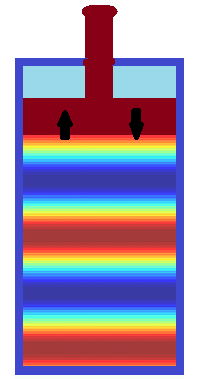On the picture above different areas of air pressure are presented in different colors from high pressure in blue to low pressure in red.

Let's introduce some wave characteristics, assuming that the piston in our experiment is periodically and reciprocally moving back and forth significantly faster than air molecules inside a cylinder can evenly distribute.
We also assume that a cylinder is long enough (strictly speaking, of infinite length, that is, bottomless), so we don't have to worry about a reflection wave from its bottom that superimposes on the primary wave initiated by a piston.

These are conditions when waves of high and lower pressure are following each other with constant frequency and with constant distance between the peaks.
The first parameter that we introduce to characterize the waves is the wave length, which is a distance from one peak of pressure to the next one. We can talk about distance as the length between the peaks, that is wave length λ, and the time that passes between one wave crest passes a particular point and the next one, which is wave period T .

From these two parameters of waves we derive wave speed v=λ/T and frequency ν=1/T.

Let's represent graphically the waves in a medium in terms of pressure p as a function of time t and distance d from a piston, the source of oscillation.

In a cylinder with a piston the pressure of air is inversely proportional to the volume of a part of a cylinder under a piston, which, in turn, is proportional to a position of a piston, since cross section of a cylinder is constant.

Assume, the piston is reciprocally moving in harmonic oscillations, and its very small displacement Δy(t) relatively to some initial position can be described as a function of time t as
Δy(t) = A·sin(ω·t)
where
A is a very small amplitude of deviations from initial position and
ω=2π/T is an angular frequency of piston's oscillations.
We have chosen to use a function sin, so at time t=0 the deviation from initial position is zero.

Let's limit the oscillations to only one period (one back and forth movement of a piston) and assume that the time of a period T is very small, so we can examine the behavior of a single wave.

Let's examine the air in the area immediately under a piston - a very thin layer of molecules having initial volume V0.
We can assume that within this very thin layer the pressure of air during piston's movement is the same and only depends on its volume, which, in turn, is a function of the position of a piston. Farther down a cylinder the situation will be different since areas of high and low pressure will not travel immediately, but with some speed that depends on the property of air.

Obviously, a change of volume of a thin layer of air immediately under a piston ΔV(t) can also be represented as harmonic oscillations around initial value V0
ΔV = B·sin(ω·t)
where
B is a very small amplitude of deviations from initial volume and
ω is the same as above angular frequency of piston's oscillations.
So, the volume of our thin layer of air, as a function of time, is
V(t) = V0 + B·sin(ω·t)
where
V0 is the initial volume of our thin layer of air at time t=0.

According to Boyle's Law, air pressure p(t) in the thin layer under a piston that we consider is inversely proportional to its volume.
Therefore,
p(t) = C / V(t)
where
C is some constant.

From this follows
p(t) = C / V(t) =
= C /
[V0 + B·sin(ω·t)]

The expression above might not look like harmonic oscillation, but, actually, it's very close to it.

Firstly, it's periodic because the numerator is a constant and the denominator is a periodic function.
Secondly, it oscillates between its maximum, when the denominator at minimum (when sin(ω·t)=−1)
pmax = C / [V0 − B]

to its minimum, when the denominator at maximum (when sin(ω·t)=+1)
pmin = C / [V0 + B]

So, we do obtain oscillations of the pressure in the area immediately adjacent to a piston, just not exactly harmonic oscillations.

Knowing the pressure in the layer immediately adjacent to a piston as a function of time p(t) and the speed v of waves propagation (that depends on the amount and characteristics of air in the cylinder), we can come up with a wave function on a distance x from an oscillating piston as a function P(x,t) of both distance x and time t.

The distance x from the piston introduces a time delay τ=x/v to a wave function, so pressure at distance x will be characterized by the same function p(t) with a shift in time by τ.
P(x,t) = p(t+τ) = p(t+x/v) =
= C /
[V0 + B·sin(ω·(t+x/v))]

Graphically, this function P(x,t) looks like this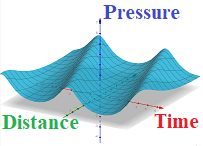As we see, the same pressure wave goes through points on any distance from the initial position of a piston, but comes there later in time.
And, the farther the point is from a piston - the later the pressure wave comes to it.

## Saturday, July 24, 2021

### Wave Equation 1: UNIZOR.COM - Physics4Teens - Waves - Waves in Medium

Notes to a video lecture on http://www.unizor.com

Wave Equation 1

In order to understand the propagation of oscillation in a medium, in the last lecture we discussed the oscillation of two molecules inside a thin rod.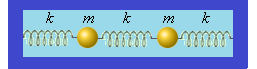We left our problem with a system of two differential equations describing the displacements x1(t) and x2(t) of these two molecules of mass m each, connected by weightless springs of elasticity k, as functions of time t:
m·x"1(t) + 2k·x1(t) − k·x2(t) = 0
m·x"2(t) − k·x1(t) + 2k·x2(t) = 0
or, using the familiar concept of angular speed ω²=k/m,
x"1(t) + 2ω²·x1(t) − ω²·x2(t) = 0
x"2(t) − ω²·x1(t) + 2ω²·x2(t) = 0

We then suggested a vector form of this system of equations that resembles the equation of harmonic oscillations.
Consider a vector X(t) of displacements
X(t) =
 x1(t) x2(t)

and a matrix Ω of coefficients
Ω =
 2ω² −ω² −ω² 2ω²

Now our system of two differential equations can be represented as one equation with vector argument X(t):
X"(t) + Ω·X(t) = 0
where X"(t) signifies a vector of second derivatives of each component of a vector X(t) and 0 signifies a null-vector.
An operation · (dot) in this equation signifies a multiplication of a matrix Ω by a vector X(t).

Compare it with an equation for harmonic oscillations we discussed in the very first lecture on Waves
x"(t) + (k/m)·x(t) = 0
where later on we assigned ω²=k/m.
Similarity of equations is obvious. Can solution be similar as well?

Multiplication of a vector by a matrix represents a linear transformation of a vector space. This transformation can make a vector longer or shorter, it can turn it to any direction, including an opposite one.

Here is a matrix that stretches any vector by a factor of 5:
 5 0 0 5

Indeed, multiplication of this matrix by a vector
 p q
results in
 5·p+0·q 0·p+5·q
=
 5·p 5·q

that is, an original vector stretched in length by a factor of 5.

Here is a matrix that turns any vector by 90°:
 0 −1 1 0

Indeed, multiplication of this matrix by a vector
 p q
results in
 0·p−1·q 1·p+0·q
=
 −q p

that is, an original vector turned counterclockwise by 90°.

There is a big difference between the two examples above.
It's much easier to solve differential equations like our
X"(t) + Ω·X(t) = 0
if matrix Ω is of "stretching" type.
Indeed, if Ω·X(t)=λ·X(t), where λ is constant, our vector equation
X"(t) + λ·X(t) = 0
can be reduced to two independent scalar equations
x1"(t) + λ·x1(t) = 0
x2"(t) + λ·x2(t) = 0
and we know how to solve them.

Unfortunately, the matrix we are dealing with in our problem of two oscillating molecules is not of a "stretching" type.
For example, a unit vector along the X-axis will be turned and unevenly stretched by multiplication by our matrix:
 2ω² −ω² −ω² 2ω²
·
 1 0
=
 2ω² −ω²

But not everything is lost.
Apparently, there are some vectors that are just stretched by this matrix. The plan now is
(a) to find all vectors that are just stretched by our matrix and
(b) try to find partial solutions to our differential equations among only these vectors.

So, we are looking for vectors
V =
 v1 v2

such that
 2ω² −ω² −ω² 2ω²
·
 v1 v2
= λ ·
 v1 v2

or
 2ω² −ω² −ω² 2ω²
·
 v1 v2
=
 λ·v1 λ·v2

or
 2ω² −ω² −ω² 2ω²
·
 v1 v2
=
 λ 0 0 λ
·
 v1 v2

or
 (2ω²−λ) −ω² −ω² (2ω²−λ)
·
 v1 v2
=
 0 0

We cannot be satisfied with a trivial solution of null-vector v1=v1=0 to this system of equations.
To have non-trivial solutions, that is to find non-zero vectors that are stretched by our matrix, the determinant of this matrix must be equal to zero.
So, let's find λ that brings the determinant of this matrix to zero and then non-zero vectors that are stretched by this matrix.

It means, we have to solve an equation for λ:
det
 (2ω²−λ) −ω² −ω² (2ω²−λ)
= 0

or
(2ω²−λ)² − (−ω²)² = 0
or
(2ω²−λ)² = (ω²)²
or
2ω²−λ = ±ω²
from which we have two solutions:
λ1 = ω² and λ2 = 3ω²

These values are called eigenvalues of matrix Ω.

Potentially, there might be some vectors that our matrix Ω stretches by a factor λ1=ω² without any rotation and there might be some other vectors stretched by Ω by a factor of λ2=3ω².

Let's try to find these vectors because, since they are just stretched (that is, multiplied by a constant) by matrix Ω, we might easily find solutions to our system of differential equations by separating it into two independent equations, one for x1(t) and another for x2(t).

Firstly, let's find such a vector (or vectors) that matrix Ω stretches by λ1=ω².
 2ω² −ω² −ω² 2ω²
·
 v1 v2
= ω² ·
 v1 v2

It means that the result of multiplication of a matrix by a vector equals to multiplication of a factor by this vector.
Therefore,
 2ω²·v1−ω²·v2 −ω²·v1+2ω²·v2
=
 ω²·v1 ω²·v2

The above represents two separate scalar equations: 2ω²·v1−ω²·v2 = ω²·v1 and
−ω²·v1+2ω²·v2 = ω²·v2
Both equations produce
v1 = v2
which means that any vector with X-coordinate equaled to Y-coordinate is just stretched by matrix Ω.
Such a vector is called eigenvector.

In this case any vector function of time argument that looks like this
 x(t) x(t)

is an eigenvector that transformation described by matrix Ω stretches by a factor of λ1=ω².

Indeed,
 2ω² −ω² −ω² 2ω²
·
 x(t) x(t)
=
=
 2ω²·x(t)−ω²·x(t) −ω²·x(t)+2ω²·x(t)
=
=
 ω²·x(t) ω²·x(t)
= ω² ·
 x(t) x(t)

Secondly, let's find such a vector (or vectors) that matrix Ω stretches by λ2=3ω².
 2ω² −ω² −ω² 2ω²
·
 v1 v2
= 3ω² ·
 v1 v2

It means that the result of multiplication of a matrix by a vector equals to multiplication of a factor by this vector.
Therefore,
 2ω²·v1−ω²·v2 −ω²·v1+2ω²·v2
=
 3ω²·v1 3ω²·v2

The above represents two separate scalar equations: 2ω²·v1−ω²·v2 = 3ω²·v1 and
−ω²·v1+2ω²·v2 = 3ω²·v2
Both equations produce
v1 = −v2
which means that any vector with X-coordinate equaled by absolute value but opposite in sign to Y-coordinate is just stretched by matrix Ω.
All such vectors are also eigenvectors.

In this case any vector function of time argument that looks like this
 y(t) −y(t)

is an eigenvector that transformation described by matrix Ω stretches by a factor of λ2=3ω².

Indeed,
 2ω² −ω² −ω² 2ω²
·
 y(t) −y(t)
=
=
 2ω²·y(t)+ω²·y(t) −ω²·y(t)−2ω²·y(t)
=
=
 3ω²·y(t) −3ω²·y(t)
= 3ω² ·
 y(t) −y(t)

Summarizing the above, we have two sets of eigenvectors:
one that is stretched by matrix Ω by a factor of ω² and looks like
{P} =
 x(t) x(t)

where x(t) is any function of time t,
and another that is stretched by matrix Ω by a factor of 3ω² and looks like
{Q} =
 y(t) −y(t)

where y(t) is any function of time t.

All of the above analysis leads us to an opinion that we might attempt to look for solutions to a vector form of our differential equation
X"(t) + Ω·X(t) = 0
only among those vectors X(t) that belong to either set {P} of eigenvectors of matrix Ω with eigenvalue ω² or set {Q} of eigenvectors of matrix Ω with eigenvalue 3ω².

Assume, X(t){P},
that is X(t) =
 x(t) x(t)

where x(t) is some unknown function of time t.

Then Ω·X(t) = ω²·X(t) and our equation looks like
X"(t) + ω²·X(t) = 0 or
 x"(t) x"(t)
+ ω²·
 x(t) x(t)
=
 0 0

which is equivalent to one regular differential equation
x"(t) + ω²·x(t) = 0
which is an equation of harmonic oscillations with a general solution
x(t) = D·cos(ωt+φ)
where amplitude D and phase shift φ depend on initial conditions.

Assume, X(t){Q},
that is X(t) =
 y(t) −y(t)

where y(t) is some unknown function of time t.

Then Ω·X(t) = 3ω²·X(t) and our equation looks like
X"(t) + 3ω²·X(t) = 0 or
 y"(t) −y"(t)
+ 3ω²·
 y(t) −y(t)
=
 0 0

which is equivalent to one regular differential equation
y"(t) + 3ω²·y(t) = 0
which is an equation of harmonic oscillations with a general solution
y(t) = E·cos(√3ωt+ψ)
where amplitude E and phase shift ψ depend on initial conditions.

Now we have two different sets of solutions for our system of two degrees of freedom:
X(t) =
 D·cos(ωt+φ) D·cos(ωt+φ)

and
Y(t) =
 E·cos(√3ωt+ψ) −E·cos(√3ωt+ψ)

where D, E, φ and ψ can be any constants and ω²=k/m is a known characteristic of the system..

From the linearity of the differential equations involved follows that any linear combination of solutions from these two sets is also a solutions.

Therefore, a more general expression of solutions is
α·X(t) + β·Y(t)
or (dropping multipliers D and E since they, as well as multipliers α and β, are any numbers)
 α·cos(ωt+φ)+β·cos(√3ωt+ψ) α·cos(ωt+φ)−β·cos(√3ωt+ψ)

As we see, the motion of our two objects between springs is a superposition of two oscillations with different angular speeds, amplitudes and phase shifts.

Easy check shows that for any α, β, φ and ψ the above vector represents a solution to our vector differential equation
X"(t) + Ω·X(t) = 0

It remains to be proven that this expression encompasses all solutions and, therefore, can be considered as a general solution to differential equations that describe our system of two objects connected with springs, but this proof is a part of a more advanced course of differential equations and is outside of the scope of this course.

Another important part of the analysis is to find all unknown constants (α, β, φ and ψ) based on some initial conditions (displacements and velocities) of oscillating objects. It requires tedious but straight forward calculations and solving algebraic systems of equations, so we leave it anybody with inquisitive mind to do himself.

## Monday, July 19, 2021

### Waves in Medium: UNIZOR.COM - Physics4Teens - Waves - Waves in Medium

Notes to a video lecture on http://www.unizor.com

Waves in Medium

This lecture is about a different type of waves.
These waves are still mechanical oscillations in a sense that they are movements of objects with some periodicity, but the objects are not exactly the same as we studied in a previous chapter "Mechanical Oscillations".
The objects we discuss in this lecture are molecules of some substance, like air or water, or steel.

The word medium we use in a sense of "a substance regarded as the means of transmission of a force or effect", as defined in Merriam-Webster dictionary. Examples are air, water, wood, glass etc.
Basically, any substance can be a medium and transmit an oscillation from its source to some destination. The only thing that cannot be a medium in this sense is an absolute vacuum void of any force fields.

As an example of oscillation of a medium, consider a ringing bell after we strike it with a hammer.
For quite some time molecules inside this bell are oscillating, forcing to oscillate air around a bell as well, so we hear the sound.

Let's create a simplified model of this process.
First of all, we will research only a one-dimensional case.
As an example, consider a propagation of oscillation inside a thin metal rod after we strike one of its ends with a hammer.

Further consider that each molecule of a metal this thin rod is made of is connected by spring-like inter-molecular links with its left and right neighbors along a rod. If one molecule is oscillates, it will squeeze one link that attaches it to one neighboring molecule and stretches the other link, thus engaging its neighbors in oscillation.
In our one-dimensional case this model looks like this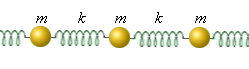where letter m indicates a molecule with its mass, while letter k indicates an elasticity of a weightless spring-like connection between molecules.

The above model of propagation of oscillation, while not perfect, allows to better understand the mechanism of waves in medium.

Finally, we simplify the problem even further by limiting the length of a rod to only two molecules, thus making the following model.In this model two identical molecules, #1 (left on the picture) and #2 (right), of mass m each are linked by identical weightless springs of length L and elasticity k among themselves and to the edges of a thin rod.
Assume that initially the left end of the rod is at the origin of X-axis, the molecule #1 is at distance L from it along the X-axis, the molecule #2 is at distance L from the first further along the X-axis and the right end of a rod is by L further down. This assumption means that initially all springs are in neutral position.
Also assume that the molecule #1 (left on the picture above) has an initial speed v, modeling a strike of a hammer on the bell.

Let x1(t) be a displacement of the molecule #1 (left on the picture above) from its initial position at moment in time t and x2(t) be a displacement of the molecule #2 (right on the picture) from its initial neutral position at time t.
Assume, the initial displacement of molecules at time t=0 is:
x1(0)=0
x2(0)=0
Their initial velocity is:
x'1(0)=v
x'2(0)=0

There are two forces acting on the molecule #1 (left on the picture above) from two springs on both sides of it, which are:
F1L(t) by the left spring attached to the left edge and
F12(t) by the middle spring attached to the molecule #2.
There are two forces acting on the molecule #2 (right) from two springs on both sides of it, which are:
F2R(t) by the right spring attached to the right edge and
F21(t) by the middle spring attached to the molecule #1.

The direction (sign) of these forces depends on the displacement of molecules, we will take it into account when constructing the equations based on the Hooke's Law.
Also, forces F12(t) by the middle spring on the first molecule and F21(t) by the middle spring on the second molecule are opposite in direction and equal by absolute value since they depend only on the length of the middle spring.

The resulting force acting on the molecule #1 is
F1(t) = F1L(t) + F12(t)
The resulting force acting on the molecule #2 is
F2(t) = F2R(t) + F21(t)

If the displacements of molecules #1 (left on the picture above) and #2 (right) at any moment of time t are, correspondingly, x1(t) and x2(t), the forces F1L(t) of the left spring and F2R(t) of the right spring, acting on molecules #1 and #2 correspondingly, according to the Hooke's Law, are
F1L(t) = −k·x1(t)
F2R(t) = −k·x2(t)

The forces of the middle spring acting on molecules #1 and #2 depend on the difference in displacements of these molecules from their neutral position.

Let
Δ(t) = x1(t) − x2(t)

If Δ(t) is positive, the middle spring is squeezed and its left end (towards molecule #1) pushes molecule #1 to the left (in negative direction), which makes F12(t) negative, while its right end pushes to the right (in positive direction) molecule #2, which makes F21(t) positive.

If Δ(t) is negative, the middle spring is stretched and its left end (towards molecule #1) pulls molecule #1 to the right (in positive direction), which makes F12(t) positive, while its right end pulls to the left (in negative direction) molecule #2, making F21(t) negative.

Therefore,
F12(t) = −k·Δ(t)
F21(t) = k·Δ(t)

Now we can express the resulting force acting on the molecule #1 is
F1(t) = −k·x1(t) − k·Δ(t) =
= −k·
[x1(t) + x1(t) − x2(t)]
And the resulting force acting on the molecule #2 is
F2(t) = −k·x2(t) + k·Δ(t) =
= −k·
[x2(t) − x1(t) + x2(t)]

Taking into account the Second Newton's Law, F1(t)=m·x"1(t) and F2(t)=m·x"2(t), we obtain the following system of two linear homogeneous differential equations of second order
m·x"1(t) = −2k·x1(t) + k·x2(t)
m·x"2(t) = k·x1(t) − 2k·x2(t)
Bringing all members to the left part of equations, we get m·x"1(t) + 2k·x1(t) − k·x2(t) = 0
m·x"2(t) − k·x1(t) + 2k·x2(t) = 0
or, using the familiar concept of angular speed ω²=k/m,
x"1(t) + 2ω²·x1(t) − ω²·x2(t) = 0
x"2(t) − ω²·x1(t) + 2ω²·x2(t) = 0

Let's approach this system of equations in a more "mathematical" way, using vectors and matrices (you can refer to "Math 4 Teens" course on UNIZOR.COM, which explains the concepts of a vector and a matrix and operations with them).

Consider a vector X(t) of displacements
 x1(t) x2(t)
and a matrix Ω of coefficients
 2ω² −ω² −ω² 2ω²

Now our system of two differential equations can be represented as one equation with vector argument
X"(t) + Ω·X(t) = 0
where X"(t) signifies a vector of second derivatives of each component of a vector X(t) and 0 signifies a null-vector.
An operation · (dot) in this equation signifies a multiplication of a matrix Ω by a vector X(t).

Compare it with an equation for a periodic movement we discussed in the very first lecture on Waves
x"(t) + (k/m)·x(t) = 0
where later on we assigned ω²=k/m.
We will try to solve a vector equation the same way we solved this similar equation in a one-dimensional case.
This is a subject of the next lecture.

## Saturday, July 3, 2021

### Forced Oscillation 2 - Resonance: UNIZOR.COM - Physics4Teens - Waves - M...

Notes to a video lecture on http://www.unizor.com

Forced Oscillation 2 -
Resonance

This lecture continues analysis of forced oscillations for a specific case, when the angular frequency of external periodic force equals to an inherent natural frequency of an object on a spring without any external forces.

Let us remind the introductory material from the previous lecture.

Oscillation is forced, when some periodic force is applied to an object that can potentially oscillate.

A subject of our analysis will be an object on a spring with no other forces acting on it except one external force that we assume is periodical.

As we know, in the absence of external force the differential equation describing the displacement x(t) from a neutral position of an object of mass m on a spring of elasticity k follows from the Hooke's Law and the Newton's Second Law
m·x"(t) = −k·x(t)
or
m·x"(t) + k·x(t) = 0
with a general solution
x(t) = A·cos(ω0·t) + B·sin(ω0·t)
where
A and B are any constants;
ω0 = √k/m is an inherent natural angular frequency of oscillation of this particular object on this particular spring.

The equation above is homogeneous because, together with any its solution x(t), the function C·x(t) will be a solution as well.

Consider a model of forced oscillation of an object on a spring with a periodic external force F(t)=F0·cos(ω·t).

Previous lecture was dedicated to a case of angular frequency of an external force ω to be different from the inherent natural frequency of an object on a spring ω0.
In this lecture we consider a case of angular frequency ω of the periodic external function F(t)=F0·cos(ω·t) to be equal to the inherent natural angular frequency ω0=√k/m of oscillations without external forces:
ω = ω0 = √k/m
So, from now on we will use only symbol ω for both inherent natural angular frequency of a spring with an object without external forces and for an angular frequency of an external force.

If external force F(t), described above, is present, the differential equation that describes the oscillation of an object looks like
m·x"(t) = −k·x(t) + F(t)
or, assuming the external force is a periodic function F(t)=F0·cos(ω·t),
m·x"(t)+ k·x(t) = F0·cos(ω·t)
where ω=√k/m

The function on the right of this equation makes this equation non-homogeneous.
Our task is to analyze the movement of an object on a spring in the presence of a periodic external function, as described by the above differential equations and some initial conditions.

Notice that if some non-homogeneous linear differential equation has two partial solutions x1(t) and x2(t), their difference is a partial solution to a corresponding homogeneous linear differential equation.
Indeed, if
m·x1"(t)+ k·x1(t) = F0·cos(ω·t)
and
m·x2"(t)+ k·x2(t) = F0·cos(ω·t)
then for x3(t)=x1(t)−x2(t)
m·x3"(t)+ k·x3(t) = 0

From the above observation follows that, in order to find a general solution to a non-homogeneous linear differential equation, it is sufficient to find its one partial solution and add to it a general solution of a corresponding homogeneous equation.

In our case we already know the general solution to a corresponding homogeneous equation
m·x"(t)+ k·x(t) = 0
So, all we need is to find a single partial solution to
m·x"(t)+ k·x(t) = F0·cos(ω·t)
where ω=√k/m
and add to it the general solution to the above homogeneous equation.

In the previous lecture, where we considered an angular frequency of external force ω to be different from the inherent natural frequency of oscillation of an object on a spring ω0, we were looking for a partial solution in a form xp(t)=C·cos(ω·t) and found such a coefficient C that the equation is satisfied:
C = F0 / [m·(ω0²−ω²)]
But, if ω=ω0=√k/m, we cannot use this method (since denominator becomes zero) and have to invent something new.

The usual recommendation in a case like this is to still use trigonometric functions, but with some simple function as a multiplier.
Let's try the following function:
xp(t) = Q·t·sin(ω·t)

Let's find second derivative of xp(t), substitute xp(t) and x"p(t) into our differential equation and find suitable values for a constant Q. That would deliver a partial solution to our non-homogeneous differential equation.

x'p(t) =
= Q·sin(ω·t) + Q·t·ω·cos(ω·t)

x"p(t) = Q·ω·cos(ω·t)+
+Q·ω·cos(ω·t)−Q·t·ω²·sin(ω·t)

Our original equation
m·x"(t)+ k·x(t) = F0·cos(ω·t)
can be written as
x"(t) + (k/m)·x(t) =
= (F0/m)·cos(ω·t)

or
x"(t)+ω²·x(t) = (F0/m)·cos(ω·t)

Substitute the partial solution expression into the left part of the differential equation above:
Q·ω·cos(ω·t)+Q·ω·cos(ω·t)−
−Q·t·ω²·sin(ω·t)+Q·t·ω²·sin(ω·t)

Canceling positive and negative terms that are equal by absolute value, got the following equation with the proposed solution
2Q·ω·cos(ω·t) =
= (F0/m)·cos(ω·t)

from which
Q = F0/(2m·ω)
The partial solution to our non-homogeneous differential equation is
xp(t) = F0·t·sin(ω·t)/(2m·ω)

Now we can express the general solution to a differential equation that describes the forced oscillation by a periodic external function as
x(t) = F0·t·sin(ω·t)/(2m·ω) +
+ A·cos(ω·t) + B·sin(ω·t)

where
A and B are any constants determined by initial conditions x(0) and x'(0);
ω = √k/m is an inherent natural angular frequency of oscillation of this particular object on this particular spring;
F0·cos(ω·t) is an external periodic force acting on an object with the same angular frequency as an inherent natural angular frequency of oscillation of this particular object on this particular spring.

It's beneficial to represent A·cos(ω·t)+B·sin(ω·t) through a single trigonometric function as follows.
Let
D = √A²+B²
Angle φ is defined by
cos(φ) = A/D
sin(φ) = B/D
(on a Cartesian coordinate plane this angle is the one from the X-axis to a vector with coordinates {A,B})
Then
A·cos(ω·t)+B·sin(ω·t) =
= D·
[cos(φ)·cos(ω·t) +
+ sin(φ)·sin(ω·t)
] =
= D·cos(ω·t−φ)

Now the general solution to our differential equation that describes forced oscillation looks like
x(t)=F0·t·sin(ω·t)/(2m·ω) +
+ D·cos(ω·t−φ)

where
F0 is an amplitude of a periodic external force;
m - mass of an object on a spring;
k - elasticity of a spring;
ω=√k/m is an inherent natural angular frequency of oscillation of this particular object on this particular spring and the angular frequency of external periodic force;
t is time;
D and φ parameters are determined by initial conditions for x(0) and x'(0).

The main component of the function describing the movement of an object, when a periodic external force acts synchronously with the inherent natural oscillation of an object on a spring, is F0·t·sin(ω·t)/(2m·ω).
This function represents oscillation with gradually, proportionally to time t, increasing to infinity amplitude.
Added to this function is a regular sinusoidal oscillation with the same angular frequency but, potentially, a phase shift φ.
The periodic external force, acting synchronously (that is, with the same angular frequency) with inherent natural oscillation of an object on a spring, causes proportional to time increasing of an amplitude of oscillations to (theoretically) infinity. This is called the resonance.

Let's represent it graphically for some specific case of initial conditions and parameters.

Initial conditions:
x(0) = 0
x'(0) = 0
From these initial conditions follows
0 = D·cos(φ)
0 = D·ω·sin(φ)
We know that ω≠0.
Also, sin(φ) and cos(φ) cannot be simultaneously equal to 0.
Therefore, D=0, and our oscillation in this case is described by a function
x(t) = F0·t·sin(ω·t)/(2m·ω)

Parameters:
m=1
k=4
ω=√k/m=2
F0=12

With the above initial conditions and parameters the oscillation of the object is described by function
x(t)=12·t·sin(2t)/(2·1·2) = 3·t·sin(2t)

The graph of this function looks like this